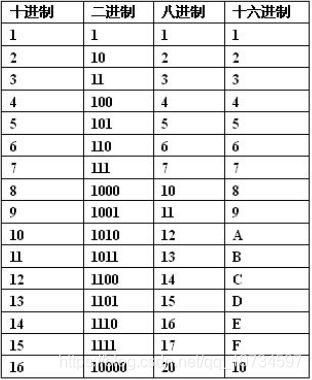# c语言十进制数字节,C语言进制转换—16进制最大能表示10进制数是多少?

1个字节：最大0xFF = 255(存储类型byte和char) 2个字节：最大0xFF FF = 65535(存储类型shot int) 4个字节：最大0xFF FF FF FF= 4294967295(存储类型int 、long) 8个字节：最大0xFF FF FF FF FF FF FF FF= 18446744073709551615(存储类型long long)16进制的20表示成10进制就是：2×161+0×16º=32

10进制的32表示成16进制就是：20

int a = 100,b = 99;

0000

0000

0000

0000

0110

0100

0×20+0×21+1×22+0×23+0×24+1×25+1×26+0×27+1×28=356

0乘以多少都是0，所以我们也可以直接跳过值为0的位：

1×22+1×25+1×26+1×28=356

4+32+64+256 =356

7×80+0×81+5×82+1×83=839

16进制就是逢16进1，但我们只有0~9这十个数字，所以我们用A，B，C，D，E，F这六个字母来分别表示10，11，12，13，14，15。字母不区分大小写。

5×160+F×161+A×162+2×163=10997

-------------------------------

10997

1234 = 1×103+2×102+3×101+4×100

1111 = 8 + 4 + 2 + 1 = 15 =F

1110 = 8 + 4 + 2 + 0 = 14= E

1101 = 8 + 4 + 0 + 1 = 13= D

1100 = 8 + 4 + 0 + 0 = 12 =C

1011 = 8 + 0 + 2 + 1 = 11= B

1010 = 8 + 0 + 2 + 0 = 10 =A

1001 = 8 + 0 + 0 + 1 =9 =9

……

0001 = 0 + 0 + 0 + 1 = 1= 1

0000 = 0 + 0 + 0 + 0 = 0= 0

1111 1101 ， 1010 0101 ， 1001 1011

F D ， A 5 ， 9 B

1234 1234/16 77 2

77 77/16 4 13 (D)

4 4/16 0 4

0100

1101

0010

0100 -- 4

1101 -- D

0010 -- 2

01101101

11100101

10101111

00011011

487710÷16=30481....14(E)

30481÷16=1905....1

1905÷16=119....1

119÷16=7....7

7÷16=0....7

C C++的表达方法

C，C++规定，16进制数必须以 0x开头。比如 0x1表示一个16进制数。而1则表示一个十进制。另外如：0xff,0xFF,0X102A，等等。其中的x也不区分大小写。(注意：0x中的0是数字0，而不是字母O)

int a = 0x100F;

int b = 0x70 + a;

\'?\' //直接输入字符

\'\77\' //用八进制，此时可以省略开头的0

\'\0x3F\' //用十六进制

06-073413
01-17502

05-2098
08-123992
05-194975
05-06844
02-26962
05-21192
05-18133
11-063288
04-15677
11-183044
08-249689
05-171785
10-182516
05-18379
03-161万+
09-281万+
07-051789

### “相关推荐”对你有帮助么？

•非常没帮助
•没帮助
•一般
•有帮助
•非常有帮助点击重新获取扫码支付余额充值